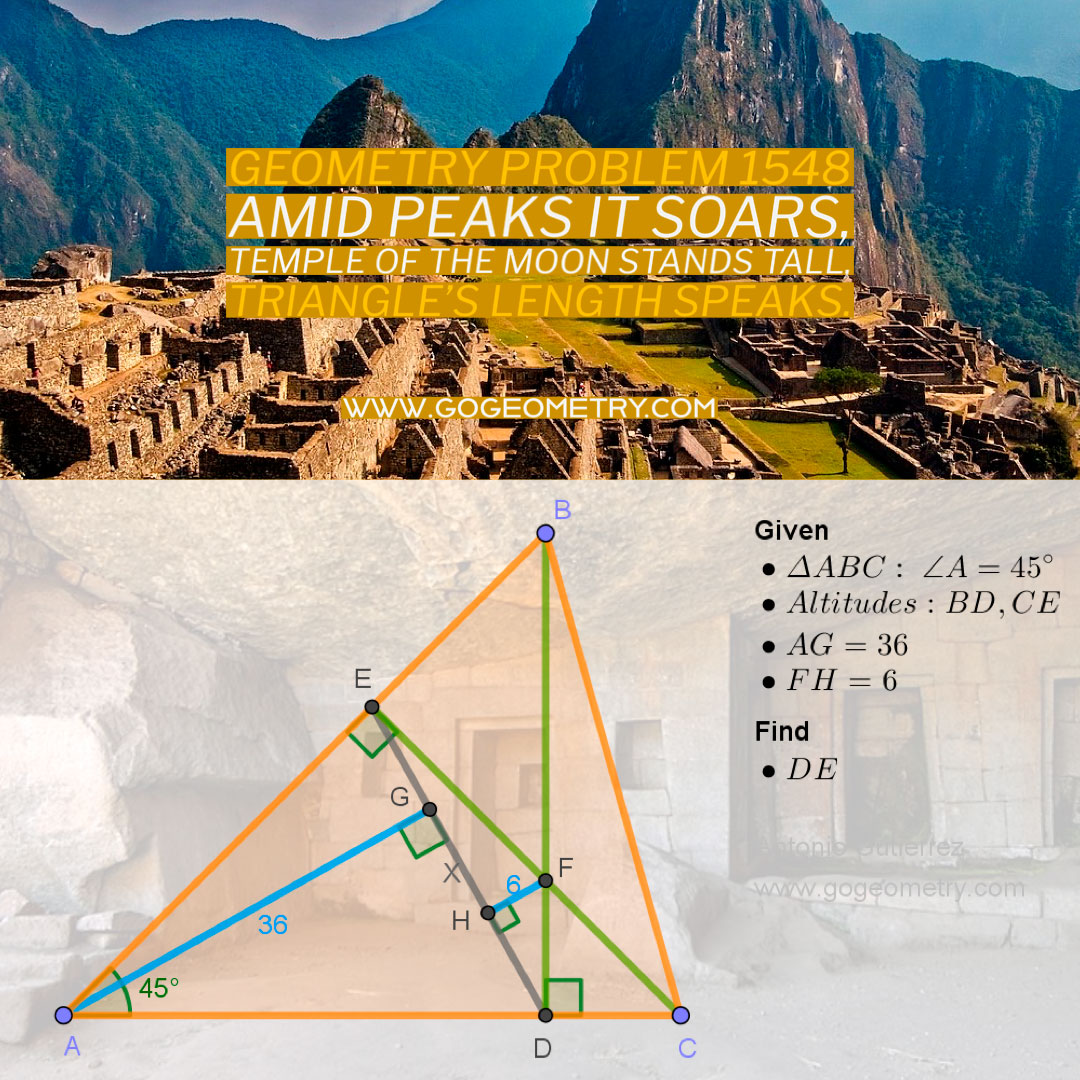# Geometry Problem 1548: Exploring Segment DE Length in Triangle ABC with a 45-Degree Angle and Intersecting Altitudes: A High School and College Level Investigation

Given a triangle ABC with angle A measuring 45 degrees, altitudes BD and CE intersect at point F. If the distance from vertex A to segment DE is 36 units and the distance from point F to segment DE is 6 units, find the length of segment DE.Triangle's 45,
Altitudes, heights converge bright,
Given lengths in sight,
Seeking DE's mystery,

## Lines and Shadows: Exploring Problem 1548 with the The Temple of the Moon at Machu Picchu as the Backdrop